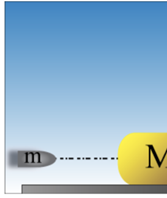# Problem: A massless spring (with force constant k = 188 N/m) connects a wall and a block of wood. The system is initially at rest, with the spring unstretched. The block has mass M = 58.5 g and is able to move without friction on a table. A gun is positioned to fire a bullet of mass m = 7.1 g into the block along the spring axis. After the gun is fired, the bullet gets embedded in the block, and the spring is compressed a maximum distance d = 0.99 m.Part (a) Find an equation for the speed of the bullet v, just before it hits the block, in terms of the variables given statement.Part (b) In meters per second, what is the speed of the bullet v before it enters the block?Part (c) What is the frequency f (in Hz) of the resulting periodic motion of the block/bullet and spring system?

###### FREE Expert Solution

Law of conservation of energy:

$\overline{){{\mathbf{K}}}_{{\mathbf{i}}}{\mathbf{+}}{{\mathbf{U}}}_{{\mathbf{i}}}{\mathbf{+}}{{\mathbf{W}}}_{{\mathbf{nc}}}{\mathbf{=}}{{\mathbf{K}}}_{{\mathbf{f}}}{\mathbf{+}}{{\mathbf{U}}}_{{\mathbf{f}}}}$

Kinetic energy:

$\overline{){\mathbf{K}}{\mathbf{=}}\frac{\mathbf{1}}{\mathbf{2}}{\mathbf{m}}{{\mathbf{v}}}^{{\mathbf{2}}}}$

Spring potential energy:

$\overline{){\mathbf{U}}{\mathbf{=}}\frac{\mathbf{1}}{\mathbf{2}}{\mathbf{k}}{{\mathbf{x}}}^{{\mathbf{2}}}}$

Momentum:

$\overline{){\mathbf{p}}{\mathbf{=}}{\mathbf{m}}{\mathbf{v}}}$

(a)

Using conservation of momentum:

mv = (m + M)v'

v' = mv/(m + M)

90% (55 ratings)###### Problem Details

A massless spring (with force constant k = 188 N/m) connects a wall and a block of wood. The system is initially at rest, with the spring unstretched. The block has mass M = 58.5 g and is able to move without friction on a table. A gun is positioned to fire a bullet of mass m = 7.1 g into the block along the spring axis. After the gun is fired, the bullet gets embedded in the block, and the spring is compressed a maximum distance d = 0.99 m.
Part (a) Find an equation for the speed of the bullet v, just before it hits the block, in terms of the variables given statement.
Part (b) In meters per second, what is the speed of the bullet v before it enters the block?
Part (c) What is the frequency f (in Hz) of the resulting periodic motion of the block/bullet and spring system?